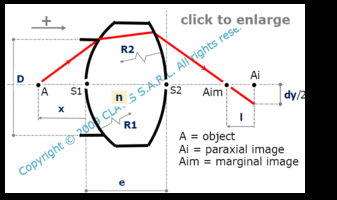and orgeometrical optics
spherical aberration

# spherical aberration of a spherical lens

Caution
Fill in all input cells.
Choose all parameters unit.
n can be either a refraction index value or the reference of a glass in the catalog.
If a refraction index value is directly entered, the wavelength will not be considered.
e is positive or null.
Raddii and x are algebric or infinite. Enter "infinite" for a plane surface or an object at infinity.
Positive direction is according the horizontal arrow in the bottom right of the scheme.

InputValueunit
R1
R2
D
e
n
x
OutputValueUnit
lto be calculated
dyto be calculatedR1 : radius of curvature of the first surface R2 : radius of curvature of the second surface D : clear aperture diameter of the dioptrum n : refraction index of the lens x : distance from the apex of the first surface to the object

Note
This calculation page can not detect all unrealistic inputs.